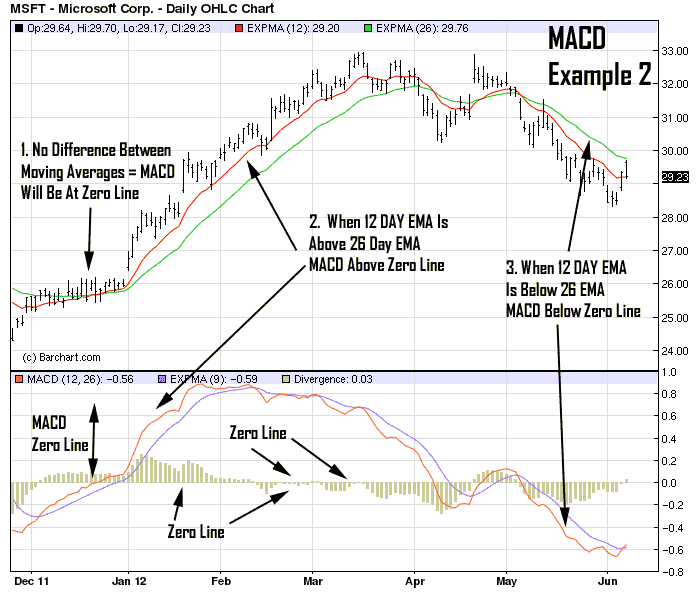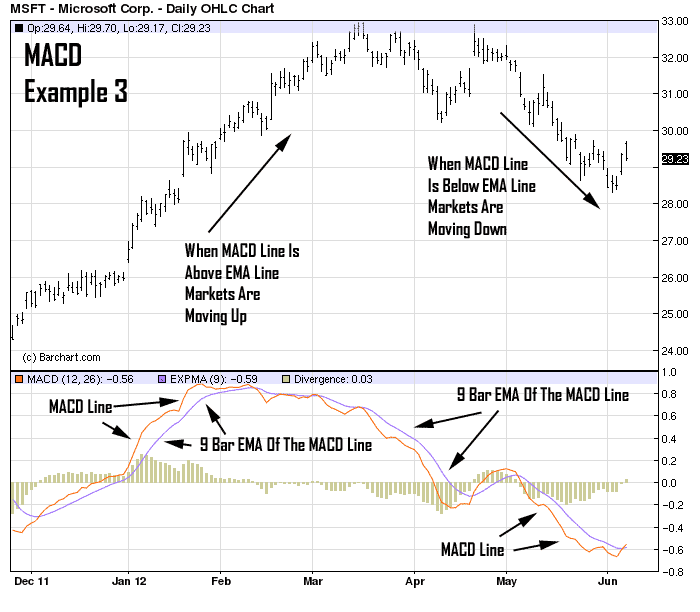# MACD Trading Tactic For Beginners

Today I’m going to introduce you to the MACD trading indicator. The MACD indicator is a trend momentum indicator that’s basically a refinement of the two moving averages and measures the distance between the two moving average lines. MACD is an acronym for Moving Average Convergence Divergence.

The indicator was developed by Gerald Appel and is discussed in his book, The Moving Average Convergence Divergence Trading Method. Needless to say over 30 years later, the MACD remains one of the most popular indicators in technical analysis.

Understanding MACD Indicator

Often time’s beginners have difficulty understanding and utilizing the MACD because it looks rather complicated and intimidating at first. Once you take the MACD apart you will realize that it’s a fairly basic indicator that is easy to understand once you familiarize yourself with it.

The MACD turns two trend-following indicators, moving averages, into a momentum oscillator by subtracting the longer moving average from the shorter moving average. As a result, the MACD offers the best of both worlds: trend following and momentum.

The MACD Is Simple Once You Understand The Basics

Three Separate Components

The first thing you should learn is the basic components that make up the MACD. You need to become familiar with these components so that the MACD graph makes sense to you. Please pay attention to this example because it lays the foundation for the next section.

The MACD is made up of three basic components. This chart shows you exactly where each of the three components are located so that you can start to see how the MACD is integrated together. The first line is the MACD line and it’s formed by subtracting the 26 bar EMA (exponential moving average) indicator from the 12 bar EMA indicator. The second line you want to pay attention to is the Signal Line.

This line is simply the 9 bar EMA of the MACD line. The purpose of this line is to smooth out the daily ups and downs of the MACD line. Finally, the last and the most visual part of the MACD is the Histogram. The Histogram is simply the difference between the MACD line and the Signal line.The MACD Is A Simple Indicator That Looks Complicated

The first thing to pay attention to is the MACD Line – This is the main component of the MACD and is calculated by subtracting the 26 period Exponential Moving Average (EMA) from the 16 period (EMA). You can see in this example how the MACD line fluctuates up and down just as soon as the Moving Averages move closer together and further apart from each other.

What I want you to understand from this example is the basic components of the MACD and how they work together. I turned on the EMA indicator for this example to make the example a bit clearer for you to understand.

There are three separate scenarios on this chart that I want you to see. First, notice when the fast and slow moving average come together on the upper part of the chart the MACD is exactly at the zero center line.

The MACD is just the difference between the two EMA’s. If there is no difference between the two then the MACD will be at the zero line. Second, when the fast 12 bar EMA moves above the 26 bar EMA the MACD moves above the zero line. Since the MACD is the difference between the two EMA’s and when the 12 bar EMA is above the 26 bar EMA a positive number is produced, the MACD will be above the zero line.

Third, when the fast 12 bar EMA is below the 26 bar EMA the difference between the two bars will be negative and will cause the MACD to move below the zero line. You can see each of these scenarios in this example.The MACD Will Hit The Zero Line When Both EMA’s Are Equal To Each Other

Use the MACD + 9,572 other factors for 2,265% gains

And not just a few, but try 9,572 other factors.

This groundbreaking system scans thousands of factors to find lightning stocks, BEFORE they strike in a matter of seconds.

Enlightened traders all across the world have now used this lightning stock blueprint to experience 981%... 1893%.. and even 2265% gains.

Want to learn this blueprint?

Signal Line Crossovers

One of the most basic MACD trading methods is Signal Line Crossovers. This method tends to work well with volatile markets that trend often such as Foreign Currencies, Technology Stocks and Volatile ETF’s.

The signal line is just a 9-day EMA of the MACD Line. As a moving average of the indicator, it slowly follows the MACD. A bullish crossover occurs when the MACD turns up and crosses above the signal line.

A bearish crossover occurs when the MACD turns down and crosses below the signal line. Once the crossover occurs, you want to make sure both lines gain as much distance apart from each other as possible. This is a good sign that momentum is continuing in the desired direction.The Signal Line Crossover Is The Most Fundamental Way To Utilize The MACD Indicator.

Conclusion

The MACD is a great indicator that’s intimidating for many beginners. Hopefully, these short tutorials will remove any reservations about using the MACD.

Roger Scott

Senior Publisher

WealthPress